What you need today You need your Atomic

• Slides: 38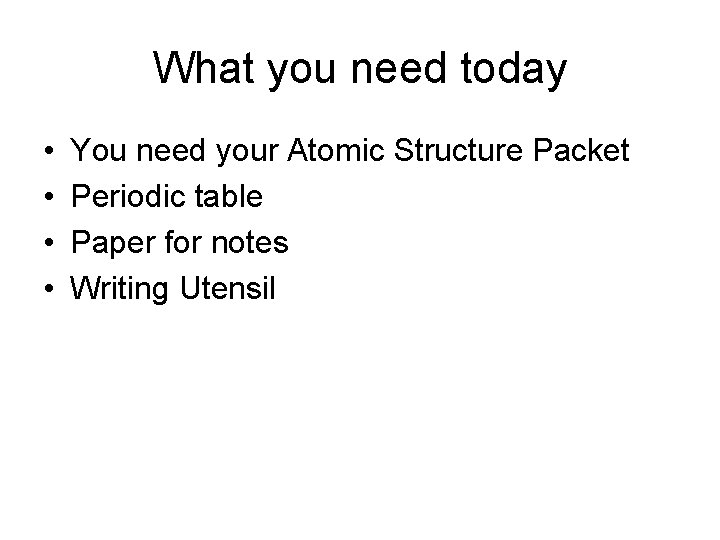What you need today • • You need your Atomic Structure Packet Periodic table Paper for notes Writing UtensilTonight’s Homework • Page 6 in Atomic Structure Packet #1 -6 orbital diagram and electron configurationVideos Watch videos 1 -8 and take notes on the handout • Click here to show videosOrbital Diagrams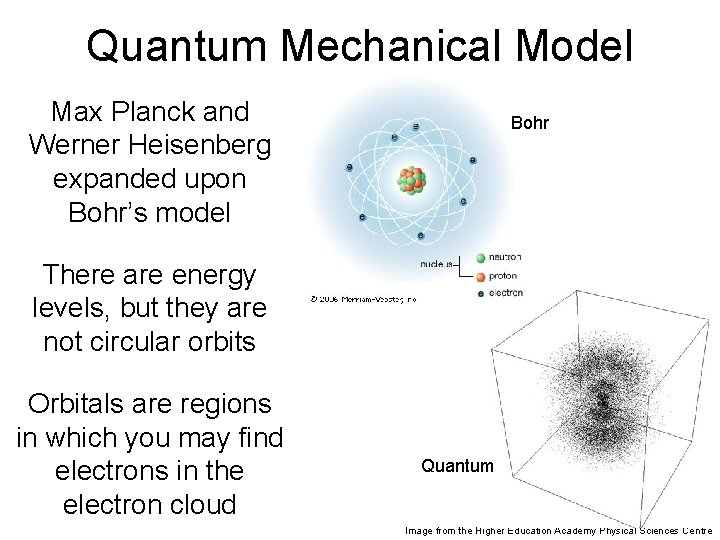Quantum Mechanical Model Max Planck and Werner Heisenberg expanded upon Bohr’s model Bohr There are energy levels, but they are not circular orbits Orbitals are regions in which you may find electrons in the electron cloud Quantum Image from the Higher Education Academy Physical Sciences Centre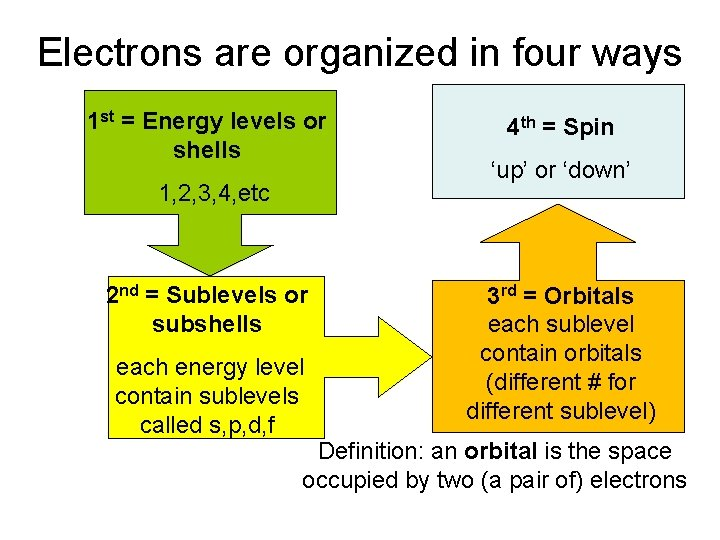Electrons are organized in four ways 1 st = Energy levels or shells 1, 2, 3, 4, etc 2 nd = Sublevels or subshells each energy level contain sublevels called s, p, d, f 4 th = Spin ‘up’ or ‘down’ 3 rd = Orbitals each sublevel contain orbitals (different # for different sublevel) Definition: an orbital is the space occupied by two (a pair of) electrons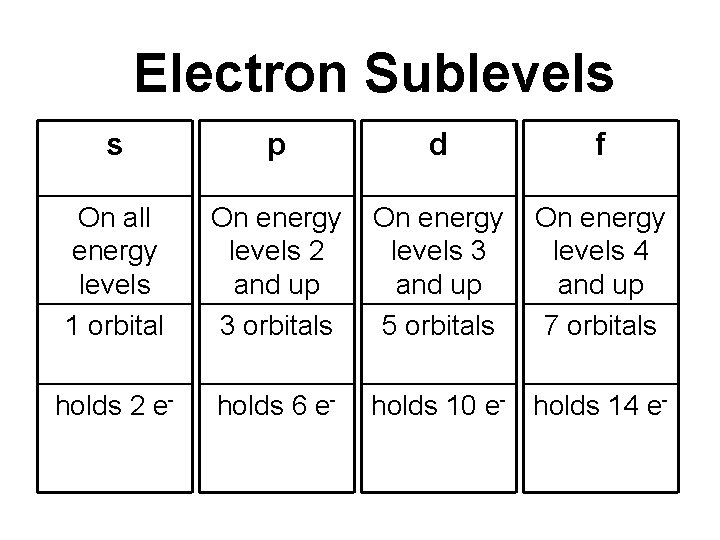Electron Sublevels s p d f On all energy levels 1 orbital On energy levels 2 and up 3 orbitals On energy levels 3 and up 5 orbitals On energy levels 4 and up 7 orbitals holds 2 e- holds 6 e- holds 10 e- holds 14 e-Practice – Rally Coach • On the next slide there a list of questions over what you just learned. • A will tell B the answer to the first question then B will tell A the answer to the next questions and so forthA: How many electrons can a d sublevel hold? B: Name the sublevels. A: What energy level does sublevel f start on? B: How many electrons can the second energy level hold? A: How many orbitals are in a p sublevel? B: How many electrons can an s sublevel hold? A: How are energy levels labeled? B: What energy level does sublevel p start on?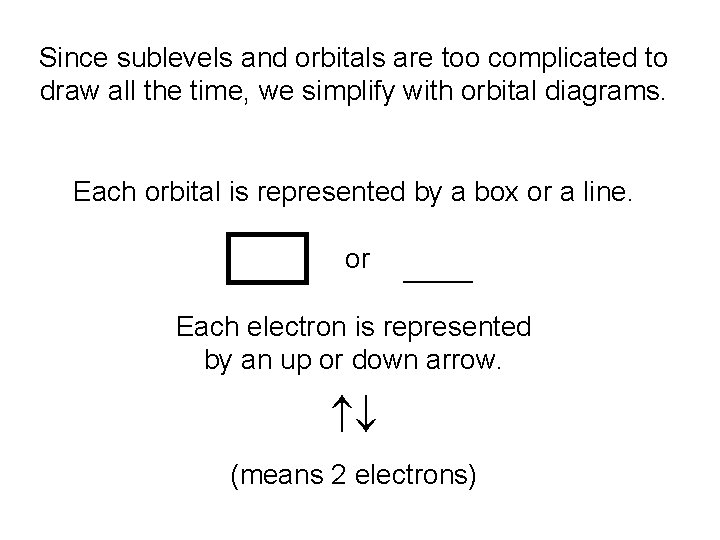Since sublevels and orbitals are too complicated to draw all the time, we simplify with orbital diagrams. Each orbital is represented by a box or a line. or _______ Each electron is represented by an up or down arrow. (means 2 electrons)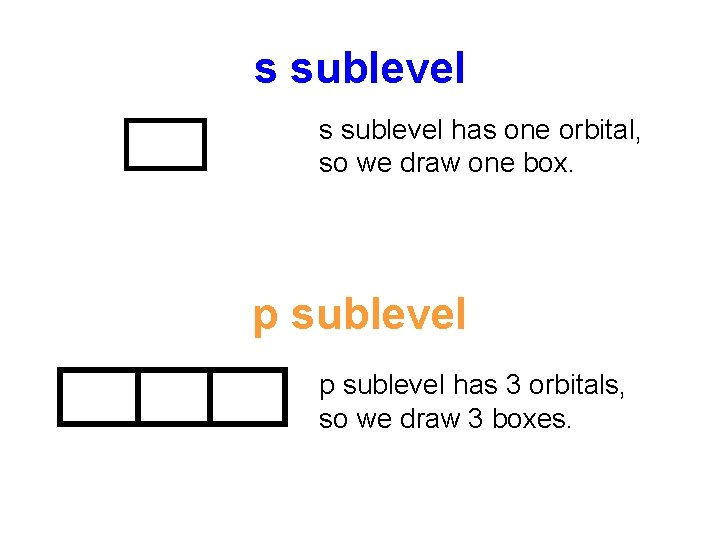s sublevel has one orbital, so we draw one box. p sublevel has 3 orbitals, so we draw 3 boxes.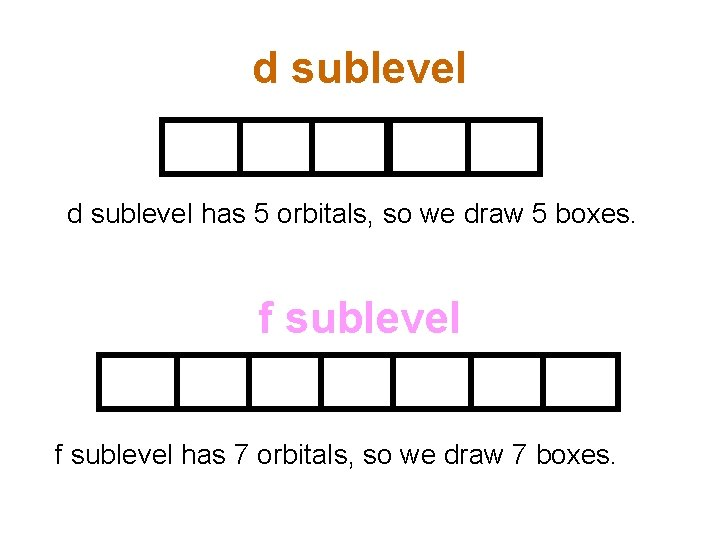d sublevel has 5 orbitals, so we draw 5 boxes. f sublevel has 7 orbitals, so we draw 7 boxes.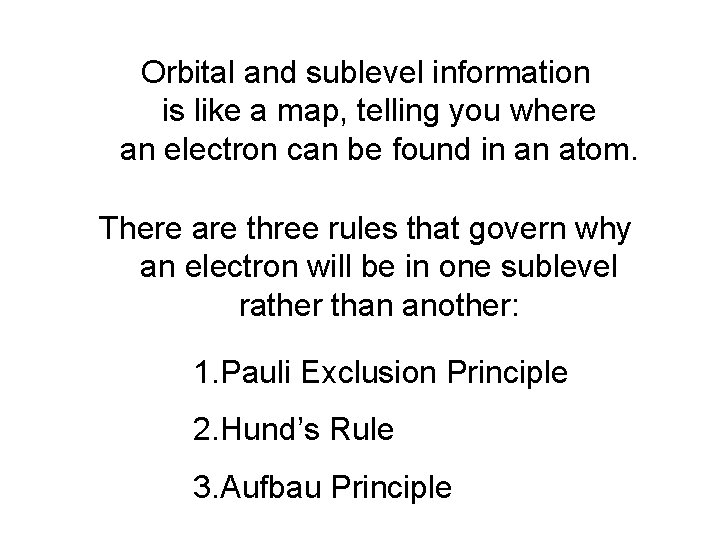Orbital and sublevel information is like a map, telling you where an electron can be found in an atom. There are three rules that govern why an electron will be in one sublevel rather than another: 1. Pauli Exclusion Principle 2. Hund’s Rule 3. Aufbau PrinciplePauli Exclusion Principle: No two electrons can be organized in exactly the same way (E. L, sublevel, orbital, spin). What that means is that 2 electrons may occupy one orbital, but they must have opposite spin direction. This is why we draw electrons as arrows facing opposite directions when they share a box: WRONG! RIGHT!Hund’s Rule of Maximum Multiplicity: Electrons occupy vacant orbitals before pairing Example: 4 electrons in a d sublevel WRONG -This is not as stable. Electrons repel each other. RIGHT -This is stable. Hund’s Rule paraphrased – spread them out before you pair them up!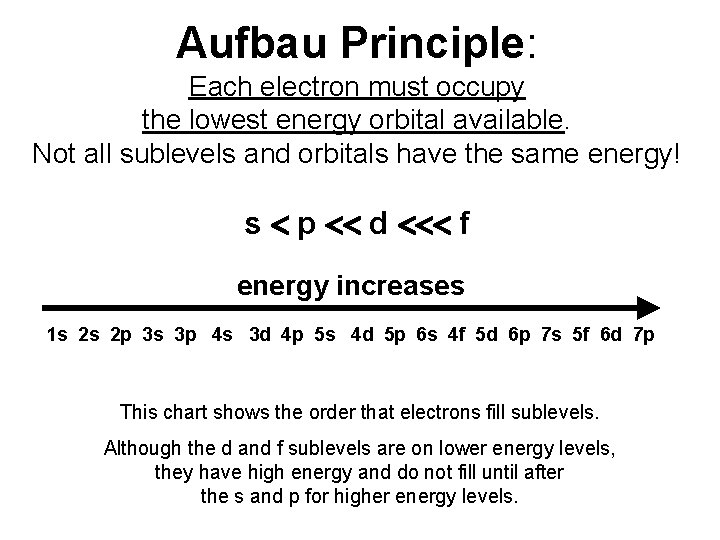Aufbau Principle: Each electron must occupy the lowest energy orbital available. Not all sublevels and orbitals have the same energy! s p d f energy increases 1 s 2 s 2 p 3 s 3 p 4 s 3 d 4 p 5 s 4 d 5 p 6 s 4 f 5 d 6 p 7 s 5 f 6 d 7 p This chart shows the order that electrons fill sublevels. Although the d and f sublevels are on lower energy levels, they have high energy and do not fill until after the s and p for higher energy levels.The number of columns in each block corresponds to the number of electrons that fit in that sublevel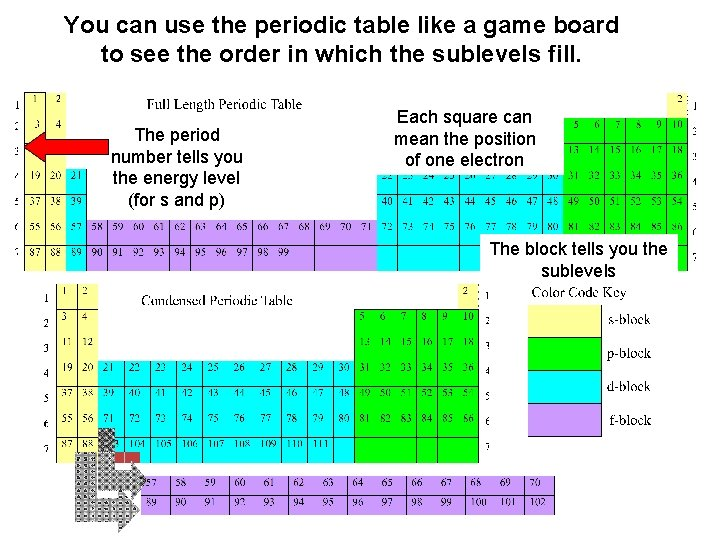You can use the periodic table like a game board to see the order in which the sublevels fill. The period number tells you the energy level (for s and p) Each square can mean the position of one electron The block tells you the sublevelsAn element’s location within the block tells you how many electrons it has in that sublevel Example: Nitrogen is in the 3 rd column of the p block. It has 3 electrons in the 2 p sublevel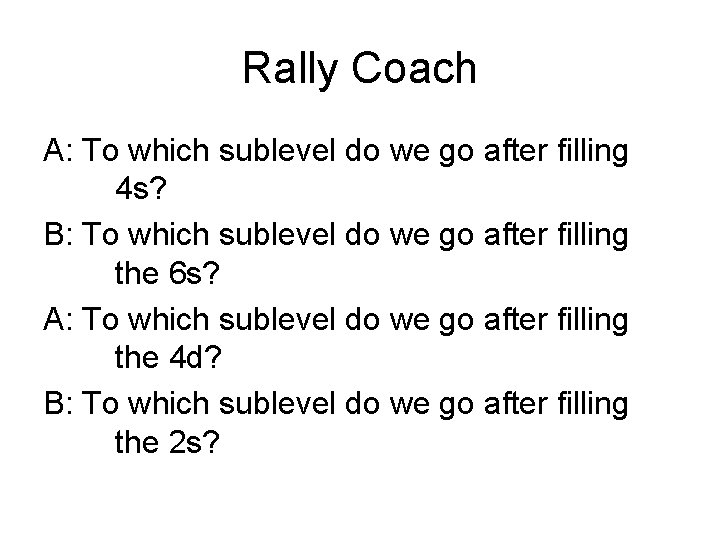Rally Coach A: To which sublevel do we go after filling 4 s? B: To which sublevel do we go after filling the 6 s? A: To which sublevel do we go after filling the 4 d? B: To which sublevel do we go after filling the 2 s?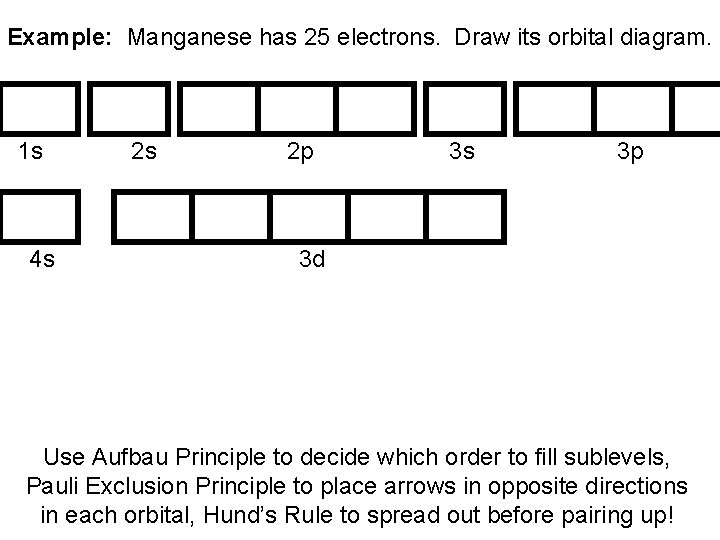Example: Manganese has 25 electrons. Draw its orbital diagram. 1 s 4 s 2 s 2 p 3 s 3 p 3 d Use Aufbau Principle to decide which order to fill sublevels, Pauli Exclusion Principle to place arrows in opposite directions in each orbital, Hund’s Rule to spread out before pairing up!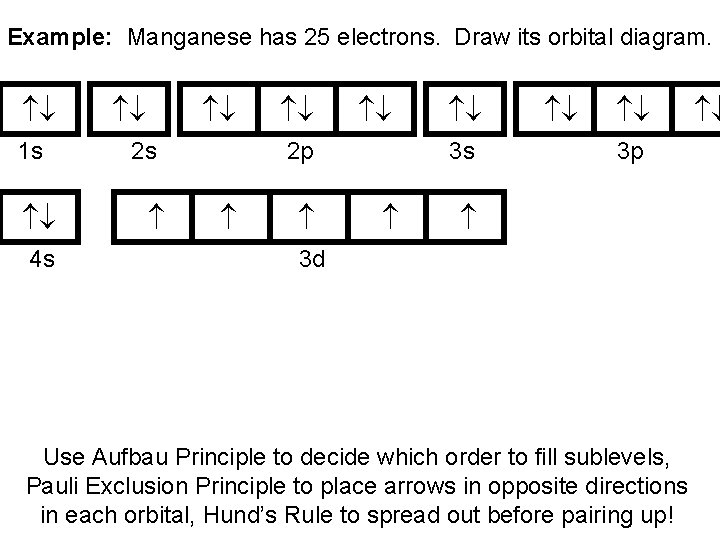Example: Manganese has 25 electrons. Draw its orbital diagram. 1 s 4 s 2 p 3 s 3 p 3 d Use Aufbau Principle to decide which order to fill sublevels, Pauli Exclusion Principle to place arrows in opposite directions in each orbital, Hund’s Rule to spread out before pairing up!Practice • Individually: write the orbital diagram for – Chlorine – Switch papers with your shoulder partner and check for accuracy (correct/celebrate) • Individually: write the orbital diagram for – Iodine – Switch papers with your face partner and check for accuracyElectron ConfigurationElectron Configurations • Draw the orbital diagram for calcium. 1 s 2 s 2 p 3 s 3 p 4 sShorthand Electron Configuration • In Aufbau order of filling electrons for Ca 1 s 2 2 p 6 3 s 2 3 p 6 4 s 2 • Superscripts are number of electrons in that sublevel • How many electrons does this neutral atom have? 1 s 2 2 p 6 3 s 2 3 p 6 4 s 1 2+2+6+1= 19 • What element is it? potassium electronsWriting Electron Configuration • Always fill electron orbitals in Aufbau Order • When you write your final electron configuration, put them in the same order electrons fill: 1 s 2 2 p 6 3 s 2 3 p 6 4 s 2 3 d 10 4 p 6 5 s 2 4 d 10 5 p 6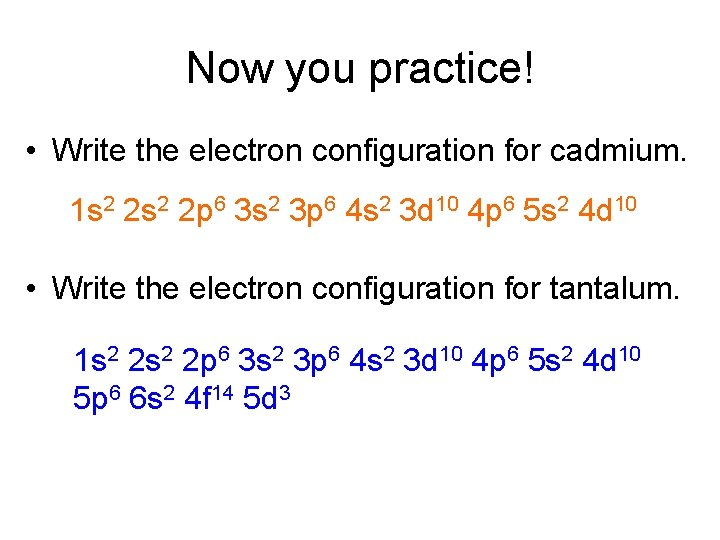Now you practice! • Write the electron configuration for cadmium. 1 s 2 2 p 6 3 s 2 3 p 6 4 s 2 3 d 10 4 p 6 5 s 2 4 d 10 • Write the electron configuration for tantalum. 1 s 2 2 p 6 3 s 2 3 p 6 4 s 2 3 d 10 4 p 6 5 s 2 4 d 10 5 p 6 6 s 2 4 f 14 5 d 3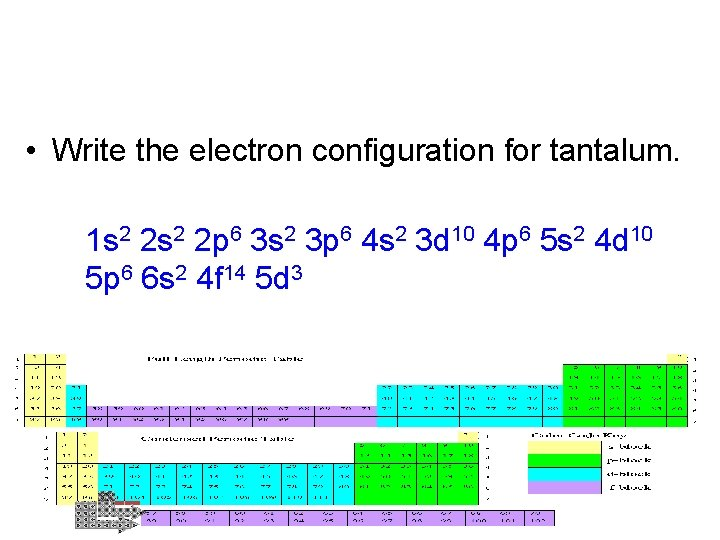• Write the electron configuration for tantalum. 1 s 2 2 p 6 3 s 2 3 p 6 4 s 2 3 d 10 4 p 6 5 s 2 4 d 10 5 p 6 6 s 2 4 f 14 5 d 3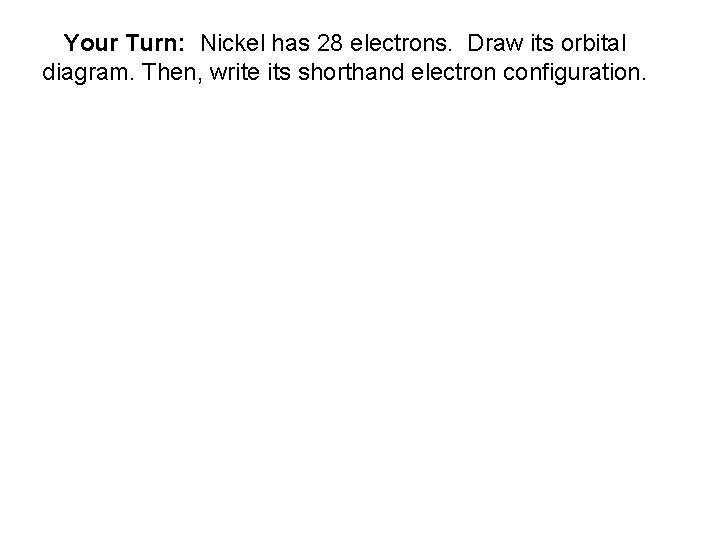Your Turn: Nickel has 28 electrons. Draw its orbital diagram. Then, write its shorthand electron configuration.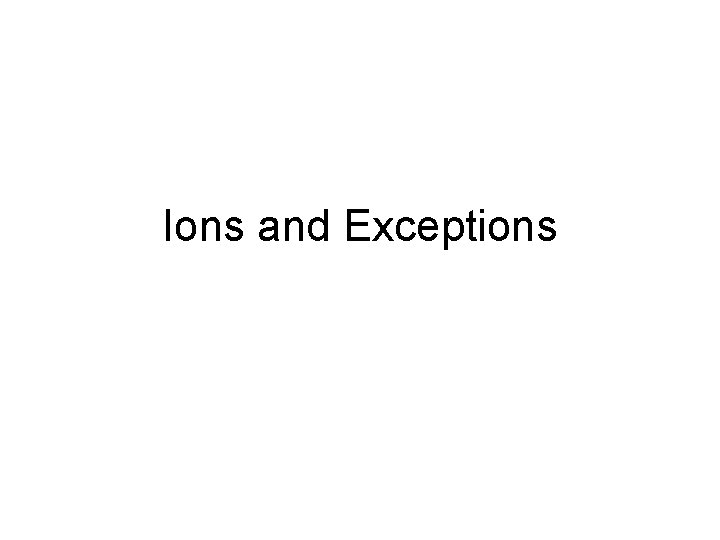Ions and Exceptions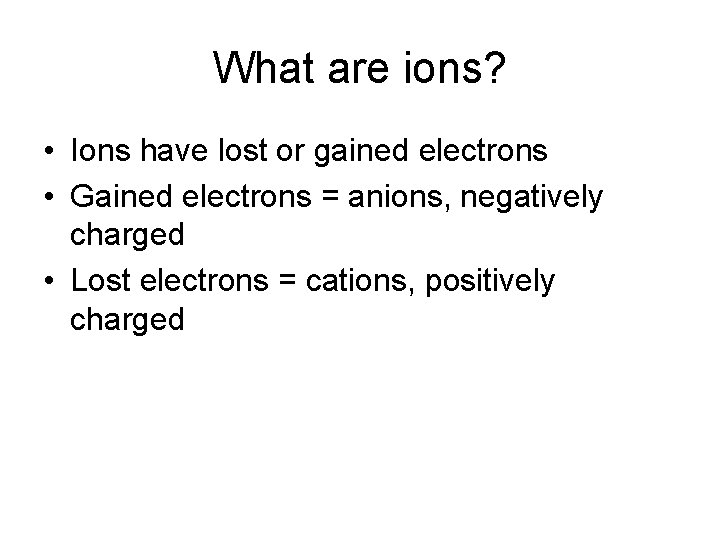What are ions? • Ions have lost or gained electrons • Gained electrons = anions, negatively charged • Lost electrons = cations, positively charged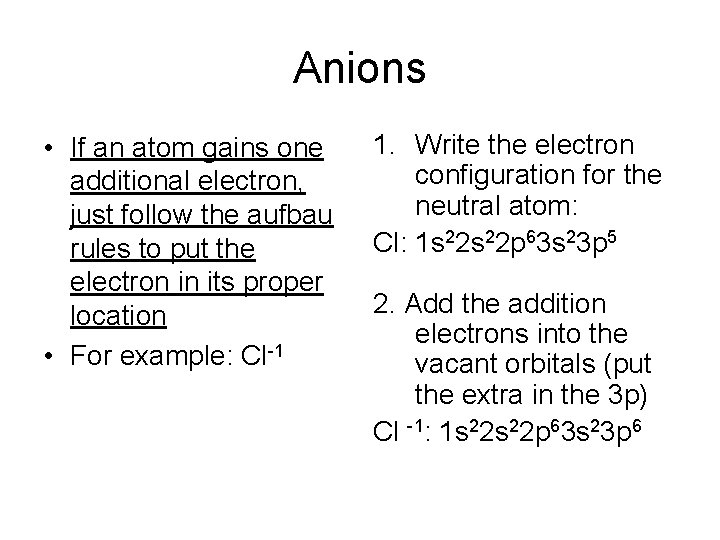Anions • If an atom gains one additional electron, just follow the aufbau rules to put the electron in its proper location • For example: Cl-1 1. Write the electron configuration for the neutral atom: Cl: 1 s 22 p 63 s 23 p 5 2. Add the addition electrons into the vacant orbitals (put the extra in the 3 p) Cl -1: 1 s 22 p 63 s 23 p 6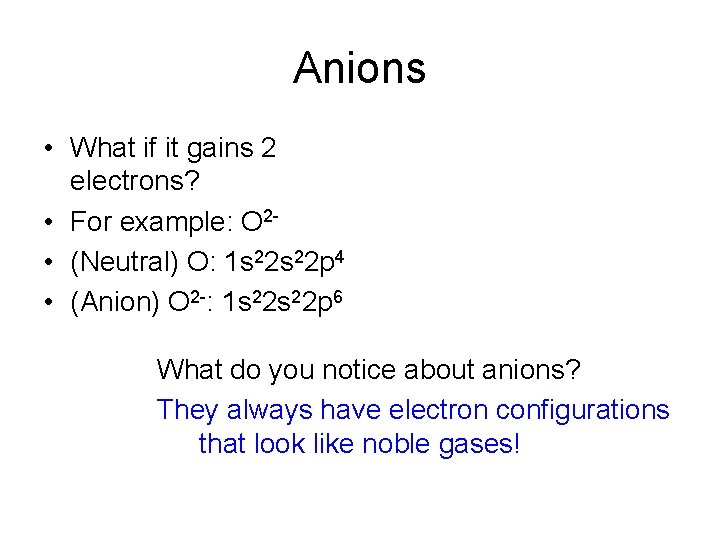Anions • What if it gains 2 electrons? • For example: O 2 • (Neutral) O: 1 s 22 p 4 • (Anion) O 2 -: 1 s 22 p 6 What do you notice about anions? They always have electron configurations that look like noble gases!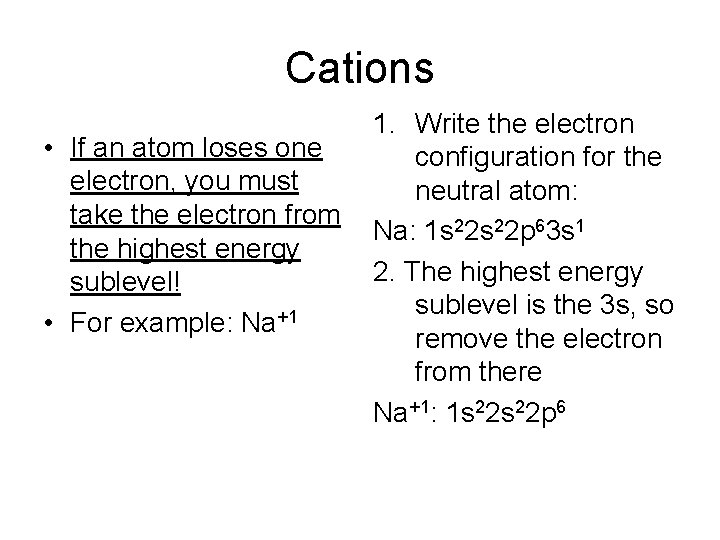Cations • If an atom loses one electron, you must take the electron from the highest energy sublevel! • For example: Na+1 1. Write the electron configuration for the neutral atom: Na: 1 s 22 p 63 s 1 2. The highest energy sublevel is the 3 s, so remove the electron from there Na+1: 1 s 22 p 6Cations • Remember, you must take the electrons from the highest energy sublevel! • For example: Ti 2+ 1. Write the electron configuration for the neutral atom: Ti: 1 s 22 p 63 s 23 p 64 s 23 d 2 2. The highest energy sublevel is the 4 s, so remove the electrons from there Ti 2+: 1 s 22 p 63 s 23 p 63 d 2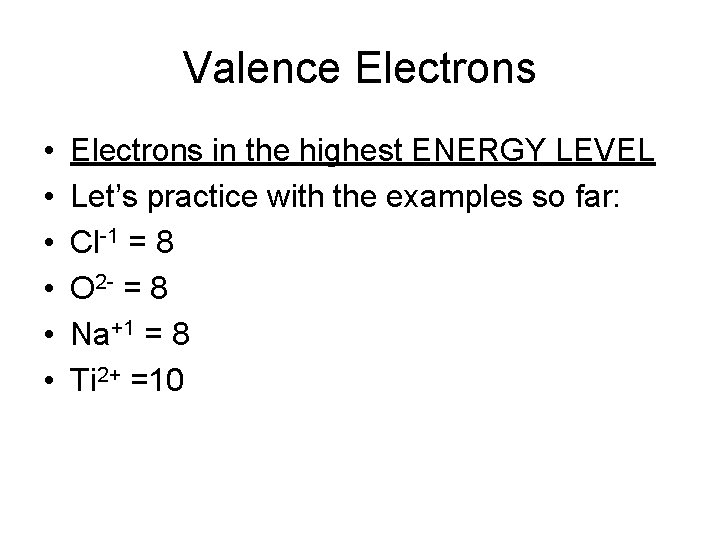Valence Electrons • • • Electrons in the highest ENERGY LEVEL Let’s practice with the examples so far: Cl-1 = 8 O 2 - = 8 Na+1 = 8 Ti 2+ =10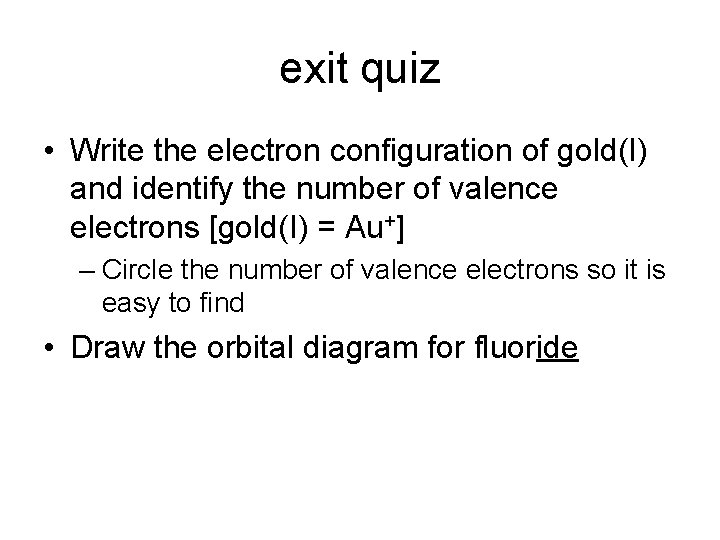exit quiz • Write the electron configuration of gold(I) and identify the number of valence electrons [gold(I) = Au+] – Circle the number of valence electrons so it is easy to find • Draw the orbital diagram for fluoride